GitHub Source

An N64 like pipeline is obviously not as complicated as any modern day graphical pipeline, but the combination of z-buffering, Gouraud shading, and texture mapping yields some pretty nice results: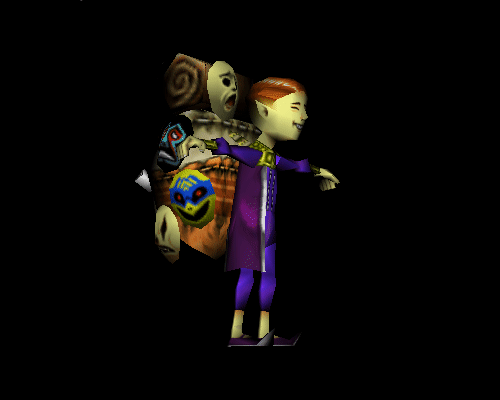This can be done in about 500 lines of pure C code without any graphic libraries. One just needs a 32 bit pointer to video memory, a strong understanding of linear algebra, and plenty of patience.

# Software renderering like it is 1996

3D models are stored in wavefront object files. These object files contain a list of vertices, faces, vertex normals, and texture vertices for each face.

Faces from the object file are centered around (0, 0, 0). To be of use the faces must be translated to fit the screen. Faces need color and are filled by wrapping a 2D rectangle around a trigon. For every pixel of the rectangle the barycentric coordinate of the trigon is calculated. If the x, y, and z coordinates of the barycenter coordinate are all greater than zero then the x and y coordinates of the rectangle are within the trigon.

The amount of light reflected from a trigon is calculated using the dot product of the sum of the trigon vertex normals with the direction of light from the camera.

Trigons in the foreground may not overlap trigons in the background. A z-buffer keeps track of pixel distance from the viewport. When a new pixel is added the z-buffer is referenced for an already drawn foreground pixel.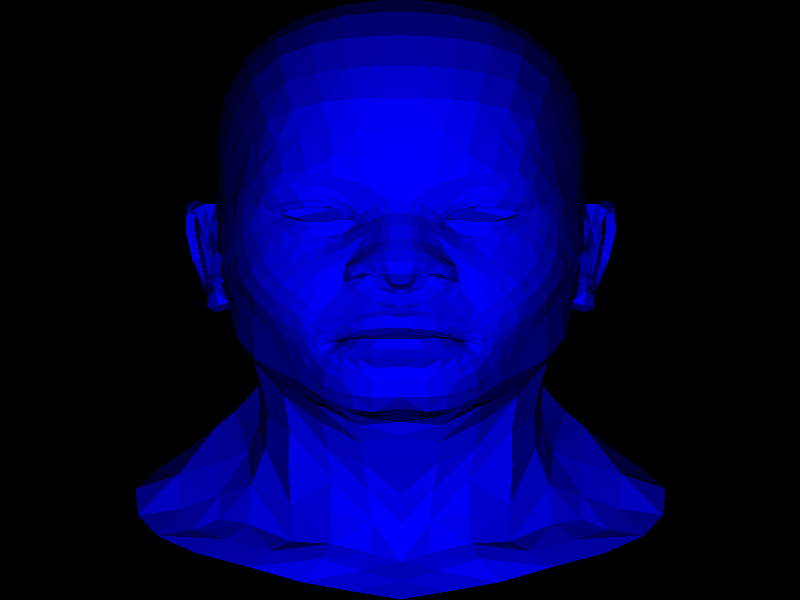A perspective view of the image is established by dividing the x and y coordinate of each vertex with the corresponding z value using (1.0 - z).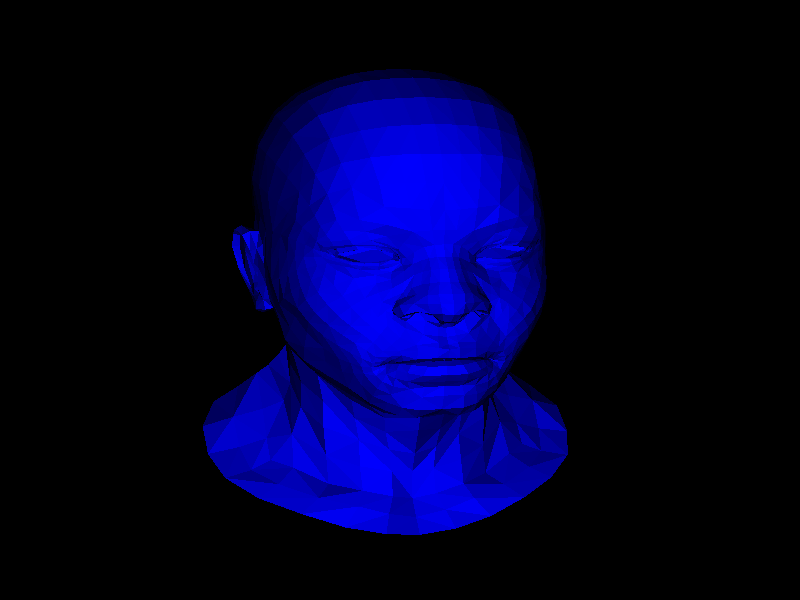By reusing the previously calculated barycenter, a varying vertex of the trigon can be calculated with dot products of the normal vertices with the direction of light from the camera. This technique is known as Gouraud shading.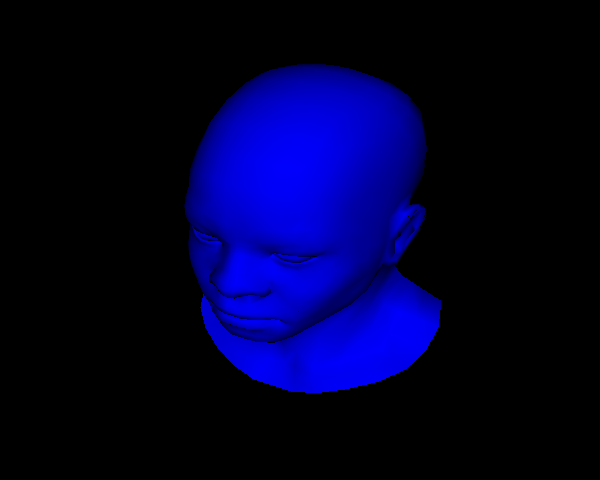By once again reusing the barycenter, an x and y coordinate can be calculated by multiplying the x, y, and z coordinates of the barycenter with the x, y, and z coordinates of the texture vertices of the trigon. This x and y coordinate will return the pixel color of the object file’s associating texture bitmap file.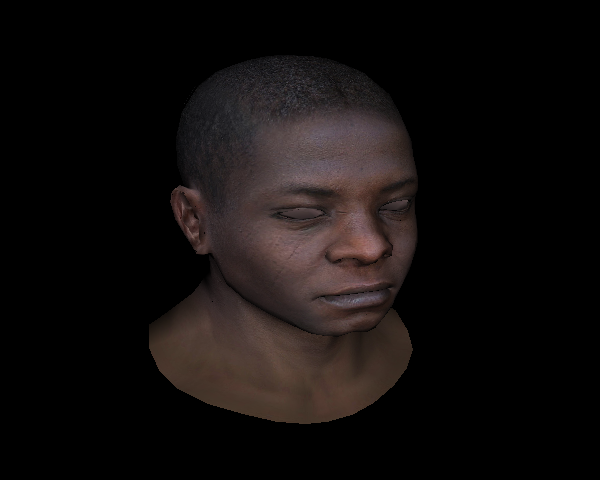And thats all there is to it. I must admit that it is easier said than done. Have a look at the implementation.